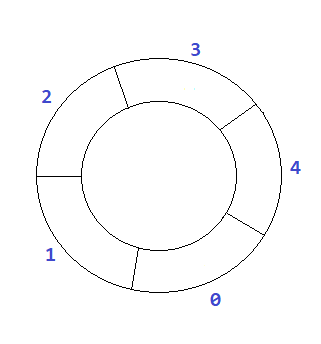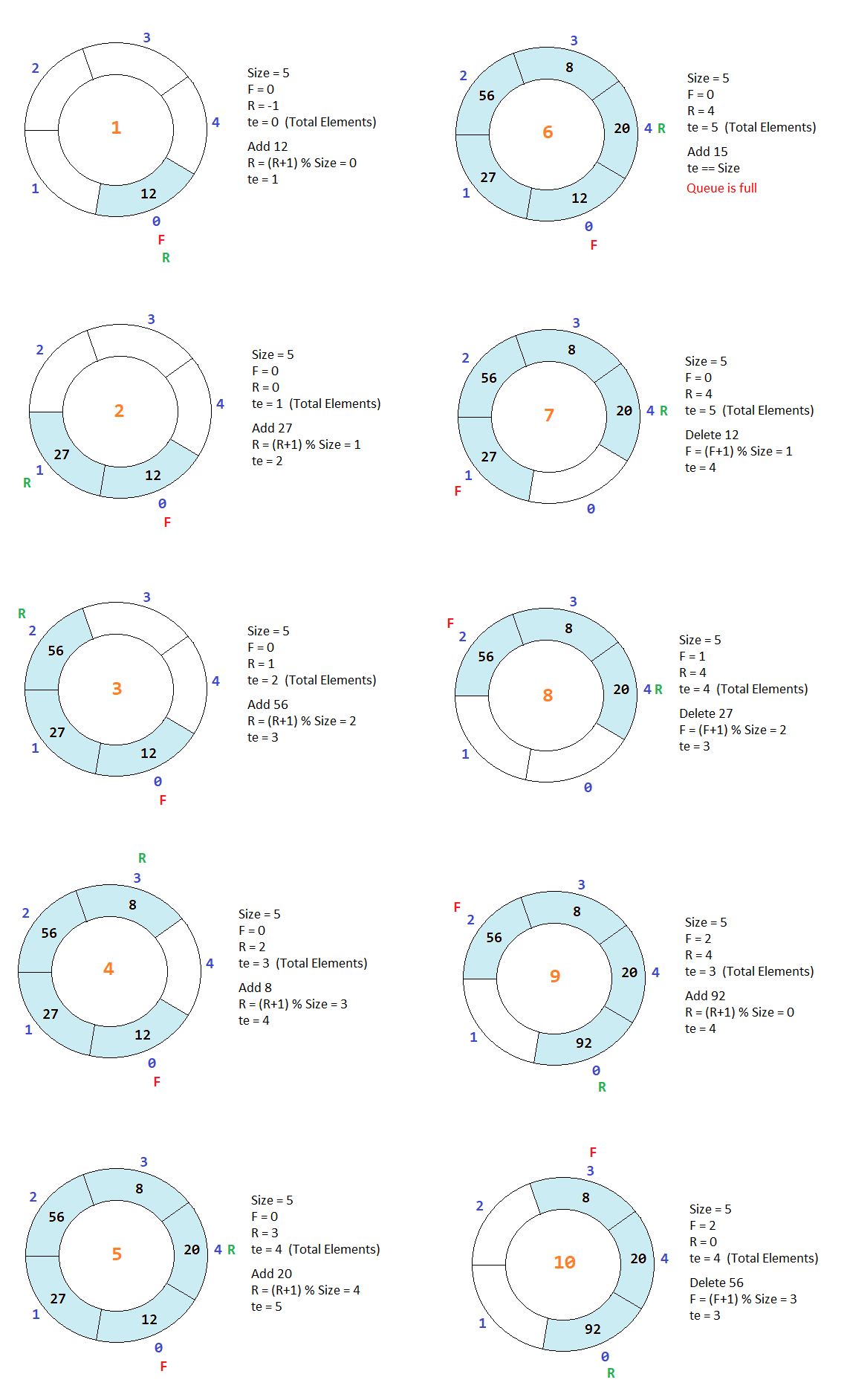# Circular Queue in Python Programming

###### User-Defined Data Structures in Python

In this lesson, we will understand what is Circular Queue in Python Programming and how to create them along with some examples.

## What is Circular Queue in Python

A Circular Queue in Python is a user-defined data structure in which elements are stored in a circular manner. In Circular Queue, after the last element, the first element occurs.

We can imagine a circular queue as given in the image below.## Operation on Circular Queue

There are two operations possible on the circular queue.

• Add - When we add an element in the circular queue.
• Delete - When we delete an element from the circular queue.

To understand how the above operations work on a circular queue. See the example given below.From the above image, we can see that when we add a new element in the circular queue, the variable R (Rare) is increased by R=(R+1)%Size, and the new element is added at the new position of R, and te is increased by 1. Similarly, when we delete an element from the circular queue, the variable F (Front) is increased by F=(F+1)%Size, and te is decreased by 1.

### Add Operation in Circular Queue

For add operation in the circular queue, first, we check if the value of te is equal to the value of size. Then, we will display a message Queue is full. Else we will increase the value of R by R=(R+1)%Size and add the element in the array at the new location of R and then increase the value of te by 1.

#### Example

``````size = 5
R = -1
F = 0
te = 0
arr = array('i',[])

if te==size:
print('Queue is full')
else:
R=(R+1)%size;
arr[R] = new_item
te = te + 1``````

### Delete Operation in Circular Queue

For the delete operation in the circular queue, first, we check if the value of te is 0. Then, we will display a message Queue is empty, else we will show the deleted element on the screen and then increase the value of F by F=(F+1)%Size and then decrease the value of te by 1.

#### Example

``````if te==0:
print('Queue is empty')
else:
print('Element Deleted = %d' %(arr[F]))
F=(F+1)%size
te = te - 1``````

## Program of Circular Queue using Array

Below is the complete program of the circular queue in Python using an array having size 5.

#### Example

``````from array import *
import os

size = 5    # Maximum numbers to be stored in the array. We can increase the quantity by changing the value 5 with a new one
arr = array('i',[])     # An empty array for queue implementation
R = -1
F = 0
te = 0

# Initilise the array with 0
for i in range(0,size):
arr.append(0)

while 1:    # An infinite while loop
os.system('cls')    # Clear the console screen in Windows OS. For Linux and MacOS use os.system('clear')
print('2. Delete')
print('3. Display')

if ch==1:
# Check if the queue is full
if te==size:
print('Queue is full')
input('Press enter to continue...')     # Pause the loop so that the user can see the above message Queue is full
else:
# If queue is not full then append the number in the array
n=int(input('Enter a number '))
R=(R+1)%size
arr[R]=n
te = te + 1

elif ch==2:
# Check if the queue is empty
if te==0:
print('Queue is empty')
input('Press enter to continue...')     # Pause the loop so that the user can see the above message Queue is empty
else:
# If queue is not empty then remove the number at index 0 from the array
print('Number Removed = %d' %(arr[F]))
F=(F+1)%size
te = te - 1
input('Press enter to continue...')     # Pause the loop so that the user can see the above message Number Removed = ?

elif ch==3:
# Check if the queue is empty
if te==0:
print('Queue is empty')
input('Press enter to continue...')     # Pause the loop so that the user can see the above message Queue is empty
else:
# if queue is not empty then display all the number
x = F
for i in range(1,te+1):
print(arr[x], end=' ')
x=(x+1)%size
input('\nPress enter to continue...')     # Pause the loop so that the user can see full queue on the screen
else:
print('Invalid Choice')
input('Press enter to continue...')     # Pause the loop so that the user can see the above message Invalid Choice``````